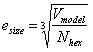# Setting up the Finite Element Model

Once the geometry to be meshed has been imported or created, the first step to defining the mesh is to set up the model. Basic parameters that are needed through the rest of the ITEM workflow are defined at this stage. Subsequent diagnostics and workflow may change based on how the model is initially set up.

Element Shape

Either a hexahedral or tetrahedral element shape may be selected. The meshing algorithm used to mesh the volumes will change based on this setting. Specific element characteristics such as the order of the element (i.e. TET10, HEX20) may be specified at a later time. The steps that will be displayed in the workflow will change based on the element type that is selected.

FEA Model Size

The number of elements or average size of the elements is an important aspect of defining your analysis model. Geometric features that are considerably smaller than the average element size, in most cases should be ignored since the mesh resolution will not be able to adequately capture them. Defining the element size at this point in the workflow permits subsequent diagnostic tests and operations to have a relative measure of what is “small”. More detailed sizing attributes such as biasing and geometry-adaptive sizing may be defined later in the ITEM workflow.

One of three different mechanisms may be used to define the size, element budget, element size and mesh density. Each of these values is dependent on the other. As a result, changing one value will automatically change the other.

• Element Budget: This value is an approximate number of elements that should be generated in the entire model. The element budget for hexahedra, Nhex, is related to the element size, esize, by the following relationship:• Where Vmodel is the geometric volume of the solid model. The element budget for tetrahedra vs. hexahedra is approximately 1:7. That is, for an equivalent edge length, a tetrahedral mesh will contain roughly seven times as many elements as a hexahedral mesh.

• Element Size: Element budget and mesh density are indirect methods for setting the element size, esize. This value can also be set explicitly. It represents the approximate average edge length of elements in the model. This size will determine the relative definition of small for subsequent diagnostic tests and will be used to set the mesh size the meshing algorithms will use.

• Mesh Density: The mesh density is represented by an integer between 1 and 10, where 1 is the finest resolution and 10 is the coarsest. It is a heuristic measure of how fine of a mesh will be generated and permits the user to indirectly set an element size without explicitly defining a real value. In most cases, the mesh density, md is related to the element size, esize by the following heuristic relationship:Where Vmax is the of the geometric volume of the largest volume in the solid model. Changing the target mesh density will display a preview of the approximate nodal spacing on the curves of the model in the graphics window.